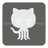8 Puzzle
Moderate
English ES PT-BR RU8 puzzle is a sliding puzzle that consists of a frame of randomly ordered, numbered square tiles with one missing tile. The object of the puzzle is to place the tiles in the right order (see picture) by using sliding moves to utilize the empty space. You can read more about this kind of puzzle on Wikipedia.

Our puzzle is presented as a 3x3 matrix with numbers from 1 to 8. Zero is the empty cell. You can "move" the empty cell in four directions: up--"U", down--"D", left--"L", and right--"R". You need to return a sequence of moves to solve...A matrix with numbers from 1 to 8 as a list of lists with integers.

The route of the empty cell as a string.

checkio([[1, 2, 3],
[4, 6, 8],
[7, 5, 0]]) == "ULDR"

The most obvious usage for the concepts in this task lie in creating a bot to solve slide puzzles; however, this task also is a fun way to learn something new because the n-puzzle is a classical problem for modeling algorithms involving heuristics.

len(puzzle) == 3
all(len(row) == 3 for row in puzzle)

You should be an authorized user in order to see the full description and start solving this mission.